# Huawei Reasoning Question

Views:5634
1. Statements :
I. All fish are traps.
II. No trap is a crack.
Conclusions :
I. No crack is a fish.
II. No fish is a crack.
1. If only conclusion I follows
2. If only conclusion II follows
3. If both conclusions follow
4. If neither of the two conclusions follows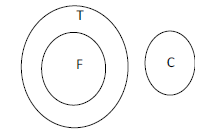So, both conclusion follow.
2. Statements :
I. All wasps are doors.
II. No door is well.
Conclusions : I. No wasp is well.
II. No well is door.
1. If only conclusion I follows
2. If only conclusion II follows
3. If both conclusions follow
4. If neither of the two conclusions follows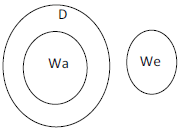So, both conclusion follow.
1. Statements:
I. All rocks are poles.
II. No pole is a horse.
Conclusions :
I. Some rocks are horses.
II. Some horses are poles.
1. If only conclusion I follows
2. If only conclusion II follows
3. If both conclusions follow
4. If neither of the two conclusions follows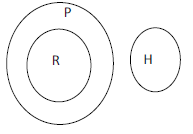So, no conclusions follow.
DIRECTIONS for questions 4:Read the information given below and answer the question that follows.
2. You have three pots of different sizes; the larger pot can contain 8 litres, the mid-size pot can contain 5 litres, and the smaller pot can contain 3 litres. The larger pot contains 8 litres milk, the other two pots are empty. You have to divide the milk into equal halves – 4 litres each, using only these three pots as stated. You can pour/transfer milk from one pot to another pot any number of times (assuming that there is no loss of milk from one pot to another pot during any transfer). What is the minimum number of transfers (x) that you need so as to divide the total milk (i.e. 8 litres) into two equal halves – 4 litres and 4 litres?
1. 4 < x < 6
2. 6 < x < 8
3. 8 < x < 10
4. 5 < x < 7Hence the answer is 7, which gets covered in second option
DIRECTIONS for questions 5: Read the information given below and answer the question that follows.
3. Harry and Fred are called Smith and Jones, but you’re not sure if it’s Harry Smith and Fred Jones, or Harry Jones and Fred Smith.
Given that two of the following statements are false, what is Harry’s surname?
I. Harry’s surname is Jones.
II. Harry’s surname is Smith.
III. Fred’s surname is Smith.
1. I and II are false
2. I and III are false
3. II and III are false
4. Not sufficient information given
Given that two statements have to be false. Since Harry has to have a surname Jones or Smith, so one and only one of I and II has to be true. So statement III has to be false. If III is false, then Fred’s surname has to be Jones. So Harry’s surname is Smith – II is true. Hence 2.
4. Statements
1. Some spaniels are not good hunters.
2. All spaniels are gentle dogs.
Conclusion(s)
I. Therefore no gentle dogs are good hunters.
II. Therefore good hunter dogs are not gentle.
Which of the statement(s) is/are conclusion(s)?
1. I only
2. II only
3. Both I and II
4. Neither I nor II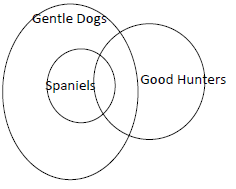Since some spaniels are not good hunters and all spaniels are gentle dogs, then some of the gentle dogs who are spaniels are not good hunters and the others are. So neither conclusion is valid.
5. Statement(s)
1. Some snakes are dangerous animals.
2. But all snakes are reptiles.
Conclusion(s)
I. Therefore some dangerous animals are not reptiles.
II. Therefore some reptiles are not dangerous animals.
1. I only
2. II only
3. Both I and II
4. Neither I nor II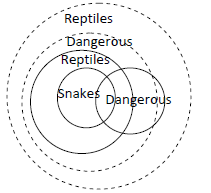Since the given statements are both affirmative, the conclusion cannot be negative. Thus neither conclusion follows.
6. Statements
1. If anything is expensive it is both valuable and rare.
2. Whatever is valuable is desirable and expensive.
Conclusion(s)
I. Therefore anything rare and expensive is desirable.
II. Therefore if anything is either valuable or expensive then it must be both valuable and expensive.
Which of the statement(s) is/are conclusion(s) ?
1. I only
2. II only
3. Both I and II
4. Neither I nor II
If all expensive are valuable and rare and all valuable are desirable and expensive so it can be related to e.g. all X are Y and all Y are X which means basically X and Y are represented by one circle only. So, all rare and expensive are desirable and all valuable or expensive are both valuable and expensive. So, we can conclude that both conclusions are true.
DIRECTIONS for questions 9-10: What should come in place of question mark (?) in the following number/alphabetic series?
7. A I L,C L P,E O T,?
1. F R X
2. F R Y
3. G R X
4. G R Y